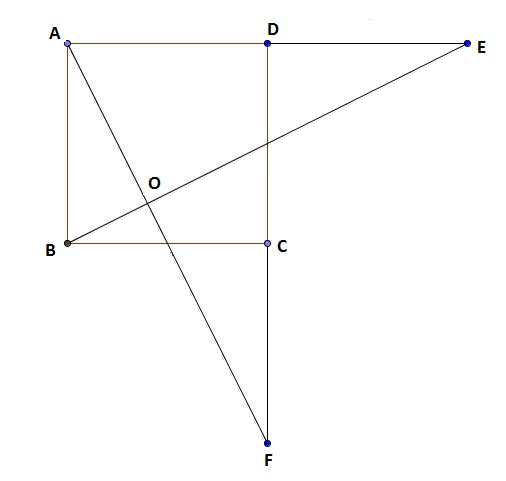# Sides Extended !!!

Geometry Level 3$ABCD$ is a square. Sides $AD$ and $DC$ are extended to $E$ and $F$ respectively such that $DE=AD$ and $CF=DC$. $BE$ and $AF$ intersect at point $O$. If $\frac{OE}{OB} = \frac{m}{n}$; where $m$ and $n$ are relatively prime, then find $m+n$.

×